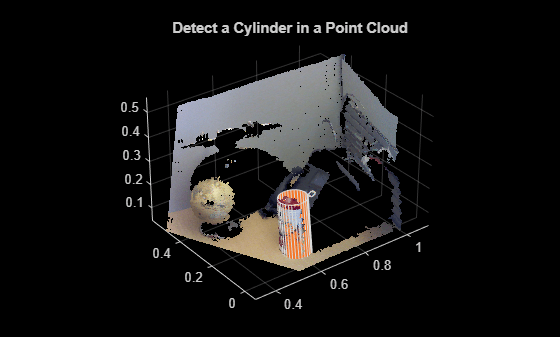Main Content

# cylinderModel class

Object for storing a parametric cylinder model

## Syntax

```model = cylinderModel(params) ```

## Description

Object for storing a parametric cylinder model.

## Construction

`model = cylinderModel(params)` constructs a parametric cylinder model from the 1-by-7 `params` input vector that describes a cylinder.

### Input Arguments

expand all

Cylinder parameters, specified as a 1-by-7 scalar vector containing seven parameters [x1,y1,z1,x2,y2,z2,r] that desribe a cylinder.

• [x1,y1,z1] and [x2,y2,z2] are the centers of each end-cap surface of the cylinder.

• r is the radius of the cylinder.

## Properties

expand all

These properties are read-only.

Cylinder model parameters, stored as a 1-by-7 scalar vector that describes a cylinder [x1,y1,z1,x2,y2,z2,r] that describe a cylinder.

• [x1,y1,z1] and [x2,y2,z2] are the centers of each end-cap surface of the cylinder.

• r is the radius of the cylinder.

Center of cylinder, stored as a 1-by-3 vector.

Height of cylinder, stored as a scalar.

Radius of cylinder, stored as a scalar.

## Methods

 plot Plot cylinder in a figure window

## Examples

collapse all

Load the point cloud.

`load('object3d.mat');`

Display point cloud.

```figure pcshow(ptCloud) xlabel('X(m)') ylabel('Y(m)') zlabel('Z(m)') title('Detect a Cylinder in a Point Cloud')```Set the maximum point-to-cylinder distance (5 mm) for the cylinder fitting.

`maxDistance = 0.005;`

Set the region of interest to constrain the search.

```roi = [0.4,0.6;-inf,0.2;0.1,inf]; sampleIndices = findPointsInROI(ptCloud,roi);```

Set the orientation constraint.

`referenceVector = [0,0,1];`

Detect the cylinder in the point cloud and extract it.

```model = pcfitcylinder(ptCloud,maxDistance,referenceVector,... 'SampleIndices',sampleIndices);```

Plot the cylinder.

```hold on plot(model)```## See Also

### Topics

Introduced in R2015b

Download ebook